# Stat Review 6: brain drain exam #2– Problems from across topic areas 6 key

 Stat Review 6: brain drain EXAM #2– Problems from across topic areas 6 KEY FORMULAS: See prior handouts STAT ESSENTIALS: See prior handouts. [NOTE: see Orange sheets for additional problems and resources.] ­­­ PROBLEMS: 1) The following table contains statistics about 200 eruptions of the Old Faithful geyser. Assume these data to be approximately normally distributed.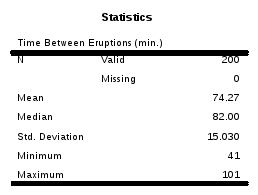1A) The time between the most recent two eruptions was 93 minutes. Determine the proportion of times between eruptions in the sample that occurred in less than 93 minutes. 1B) What time separates the quickest 10% of times between eruptions from the others? 1C) What time separates the slowest 1% of times from the rest? 1D) What proportion of the recorded times fall within +/- 2 minutes of the mean? 1A)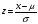=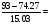1.25; area to left = .8944 DRAW A PICTURE! 1B)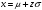= 74.27 + (-1.28)(15.03) = 55.03 minutes WHY? THE fastest times between eruptions are the shortest times - those at the left end of the distribution. So how did we get this when we do not know x or z? You are given an area from which you can obtain z. Then solve for x. 1C)= 74.27 + (2.33)(15.03) = 109.28 minutes (Same process as 1B)) 1D) Need to find the area between two values.=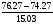= .13 => area = .5517=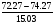= -.13 => area = .4483 => difference = .1034 2) Old Faithful revisited: 2A) Given the information provided for question #1, what is the probability that a randomly selected eruption time would exceed 100 minutes? 2B) Given the information provided for question #1, what is the probability that a randomly selected sample of 10 eruption times would exceed 100 minutes? 2C) Calculate the mean and standard deviation for all samples of size 200. 2A) Draw a picture.=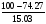= 1.72 => area = .9573 to the left 1 - .9573 = .0427, the slowest times 2B)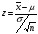=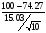= 5.41 => area to RIGHT is essentially = 0 2C) mean = population mean = 74.27 min.; standard deviation = 1.06 min. 3) Good grief, Old Faithful revisited, yet again: Referring to the data table presented for question #1, determine a 95% confidence interval for these data. 3) Confidence Interval: GIVEN: n = 200; mean = 74.27 min.; s.d. = 15.03 min.,  .05; 95% Conf. Level. From the given we find: /2 = .025; z = 1.96; large sample with sample s.d.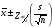=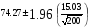= 74.27 2.08 minutes 95% C.I. = (72.19, 76.35) minutes 4) Tennis Ball Manufacturing: A company manufactures tennis balls. When its tennis balls are dropped onto a concrete surface from a height of 100 inches, the company wants the mean height of a ball’s bounce upward to be 55.5 inches. This average is maintained by periodically testing random samples of 25 tennis balls. If a 99% confidence interval contains the desired bounce height (55.5 inches), the company will be satisfied that it is manufacturing acceptable tennis balls. A sample of 25 tennis balls is randomly selected and tested. The mean bounce height of the sample is 56 inches and the standard deviation is .25 inches. Is the company making acceptable tennis balls? Explain your reasoning 4) GIVEN: n = 25; mean = 56.0 in.; s.d. = .25 in, 99% C.L. As this is a small sample, use the t formula. From the given t = 2.797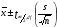=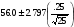=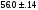=> 99% C.I. = (55.86, 56.14) So is the production meeting the desired bounce height of 55.5 inches? NO. Why not? 5) The information provided in the following tables and charts pertain to the age of cash registers and their cost of repair. Determine the level at which the correlation is significant. Identify the equation of the regression line. Assume that a cash register is 4.5 years old. Estimate the cost of repair for this cash register. Assume that the cash register is 12 years old. Estimate the cost of repair for this cash register. (“It cannot be calculated” is an incorrect answer because there is a value that can be used as the estimate.) [NOTE: In the Coefficients table under the “B,” the Constant is the y-intercept and the Age value is the slope.]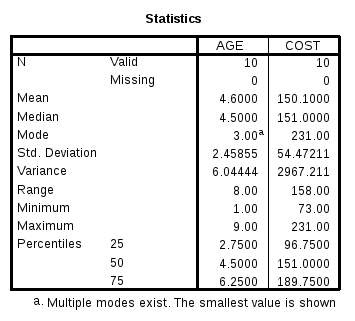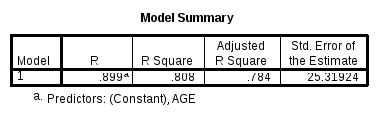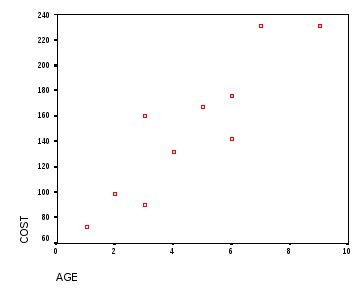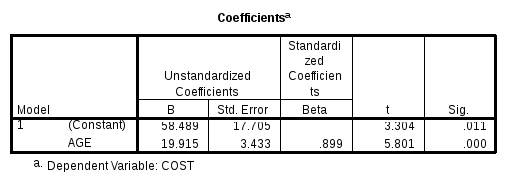Download 131.75 Kb.Share with your friends: